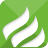﻿ MPPT Solar Controller | Why Is It Better Than the PWM-SCIENTEK ELECTRICAL

HOME NEWS MPPT Solar Controller | Why Is It Better Than the PWM

Oct 14,2020

# MPPT Solar Controller | Why Is It Better Than the PWM

Anyone who knows the solar controller knows that the controller can be divided into two types: MPPT solar controller and PWM solar controller. Of course, there are many more subdivided types. Today I will briefly talk about the difference between the two.MPPT Solar Controller

So what is the difference between the MPPT solar controller and the PWM controller? Simply put it literally, they are all controllers, but the difference lies in the two MPPT and PWM.

So what does MPPT stand for? What does PWM mean? In fact, these two can be said to be two ways of the solar controller. MPPT actually means maximum power point tracking, which can be said to be an upgrade of PWM.

While PWM is pulse modulation, the control method is to control the on and off of the switching devices of the inverter circuit, so that the output terminal gets a series of pulses of equal amplitude, and these pulses are used to replace the sine wave or the required waveform.

That is, multiple pulses are generated in the half cycle of the output waveform so that the equivalent voltage of each pulse is a sinusoidal waveform, and the obtained output is smooth and has fewer low-order harmonics. By modulating the width of each pulse according to certain rules, the output voltage of the inverter circuit can be changed, and the output frequency can also be changed.PWM Solar Controller

To put it more simply and bluntly, the conversion rate of MPPT to photovoltaic panels is relatively large, which is more than twice the conversion rate of general PWM. So the price of MPPT is naturally more expensive. We all know that there are two ways to increase the conversion rate. One is to increase the number of solar panels, and the other is to use our MPPT solar controller.

We all know that solar panels are expensive, and the MPPT solar controller improves the utilization of solar panels, so saving the number of solar panels saves money, so it is naturally more expensive.

0.195093s